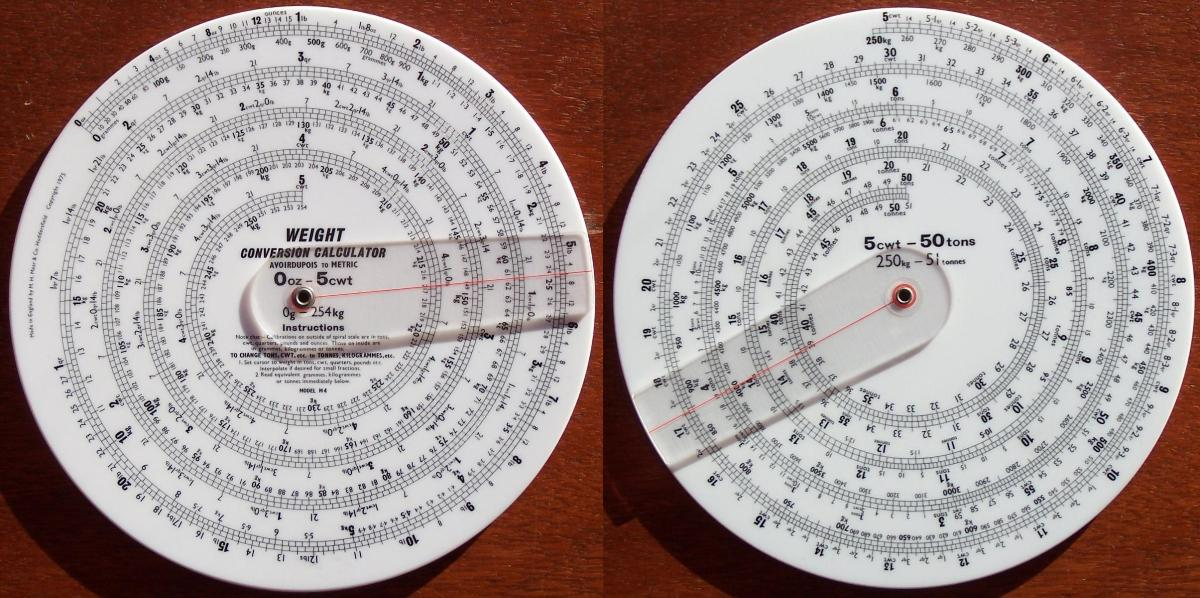## Tina's Fearn's and Mear's Calculators

 Fearn's Colliery Ventilation Officer's CalculatorMear's Converion Calculator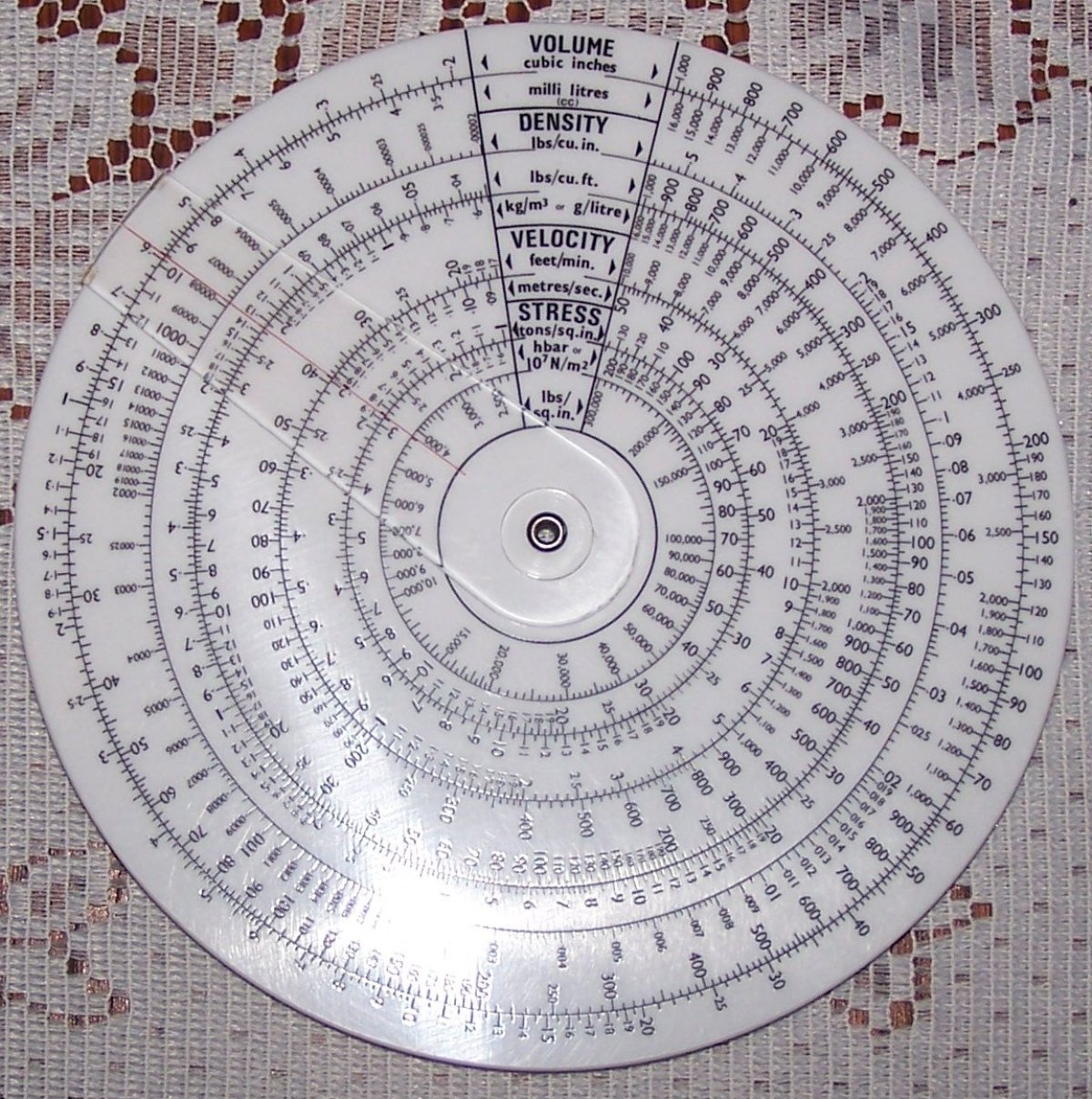Fearn's International Unit Converions CalculatorMear's Combustion Efficiency CalculatorFearn's Conveyor Belt Calculator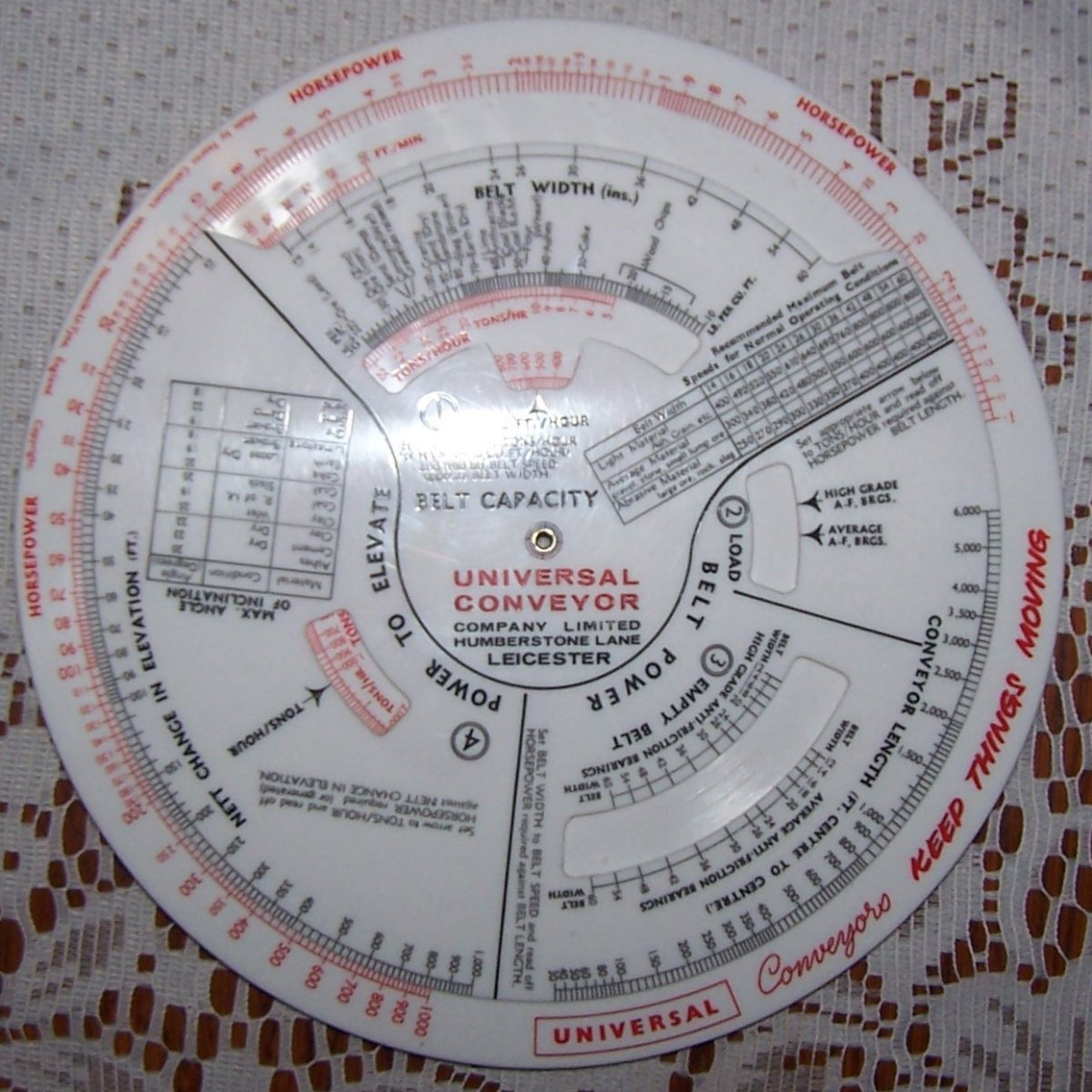Fearn's Conveyor Belt Calculator, Metric VersionFearn's Cyclone Soundrater for Centrifugal FansMear's Domestic Central Heating Calculator Imperial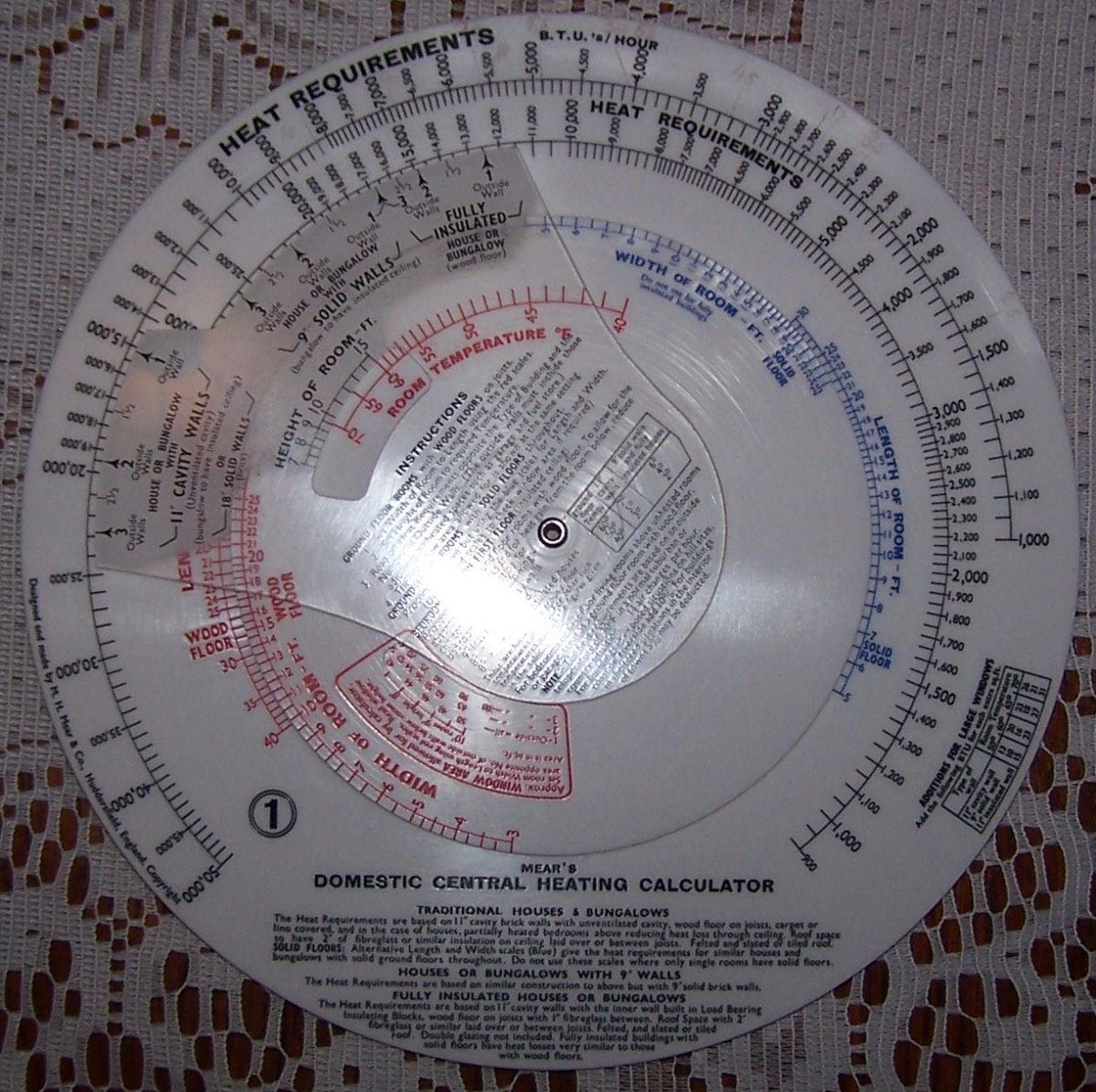Mear's Domestic Central Heating Calculator Imperial for Houses and Bungalows, Model N0.8.Mear's Industrial Central Heating Calculator, Model No.3.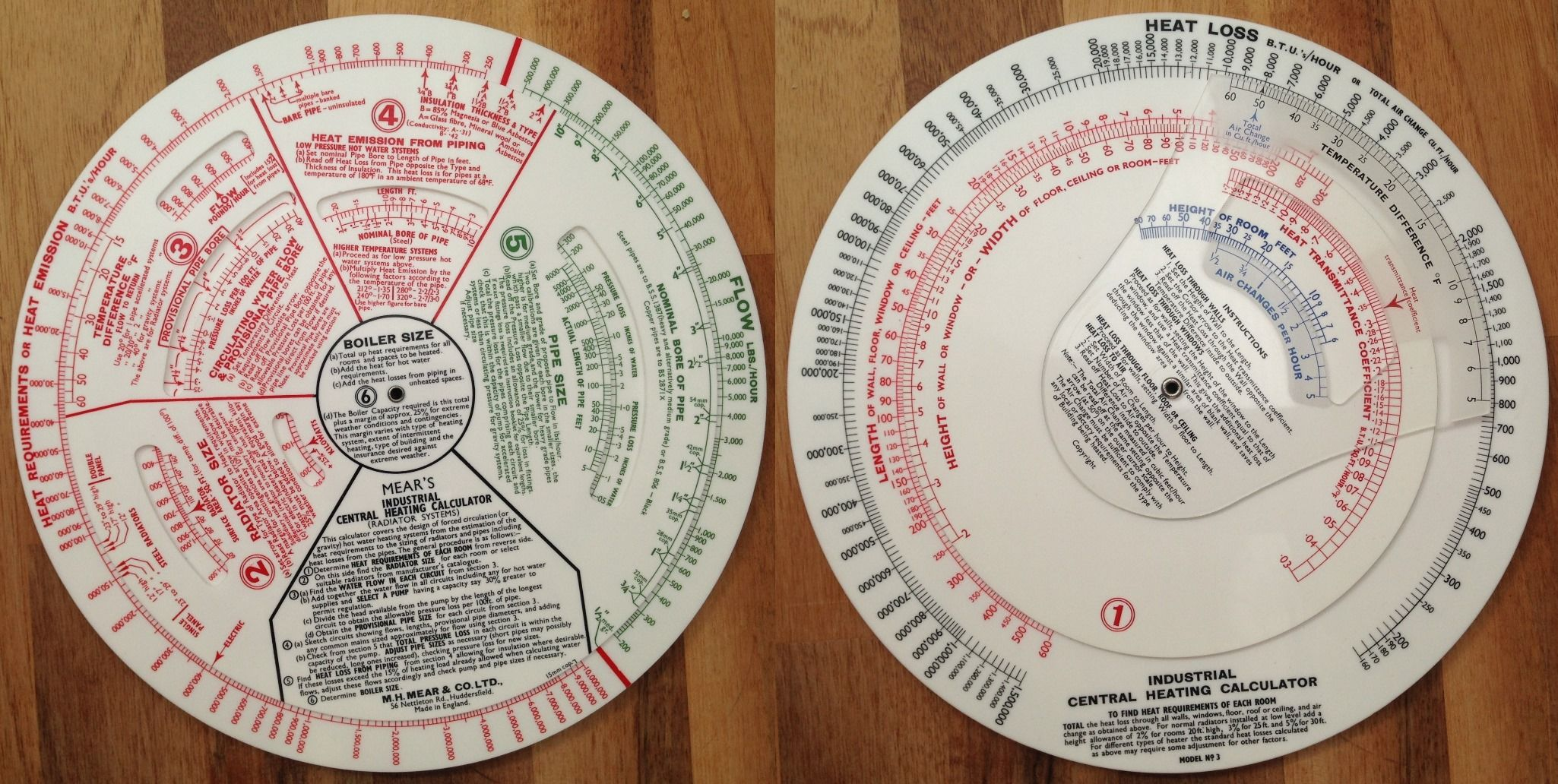Fearn's Mear Heating Engineer's Calculator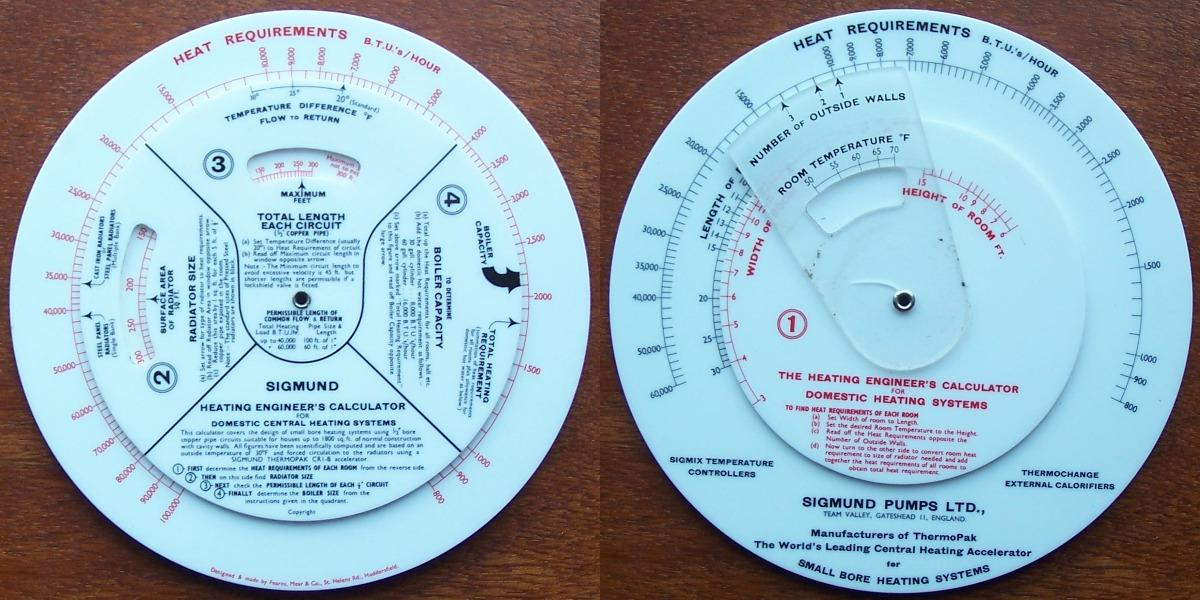Mear's Ducting Calculator Imperial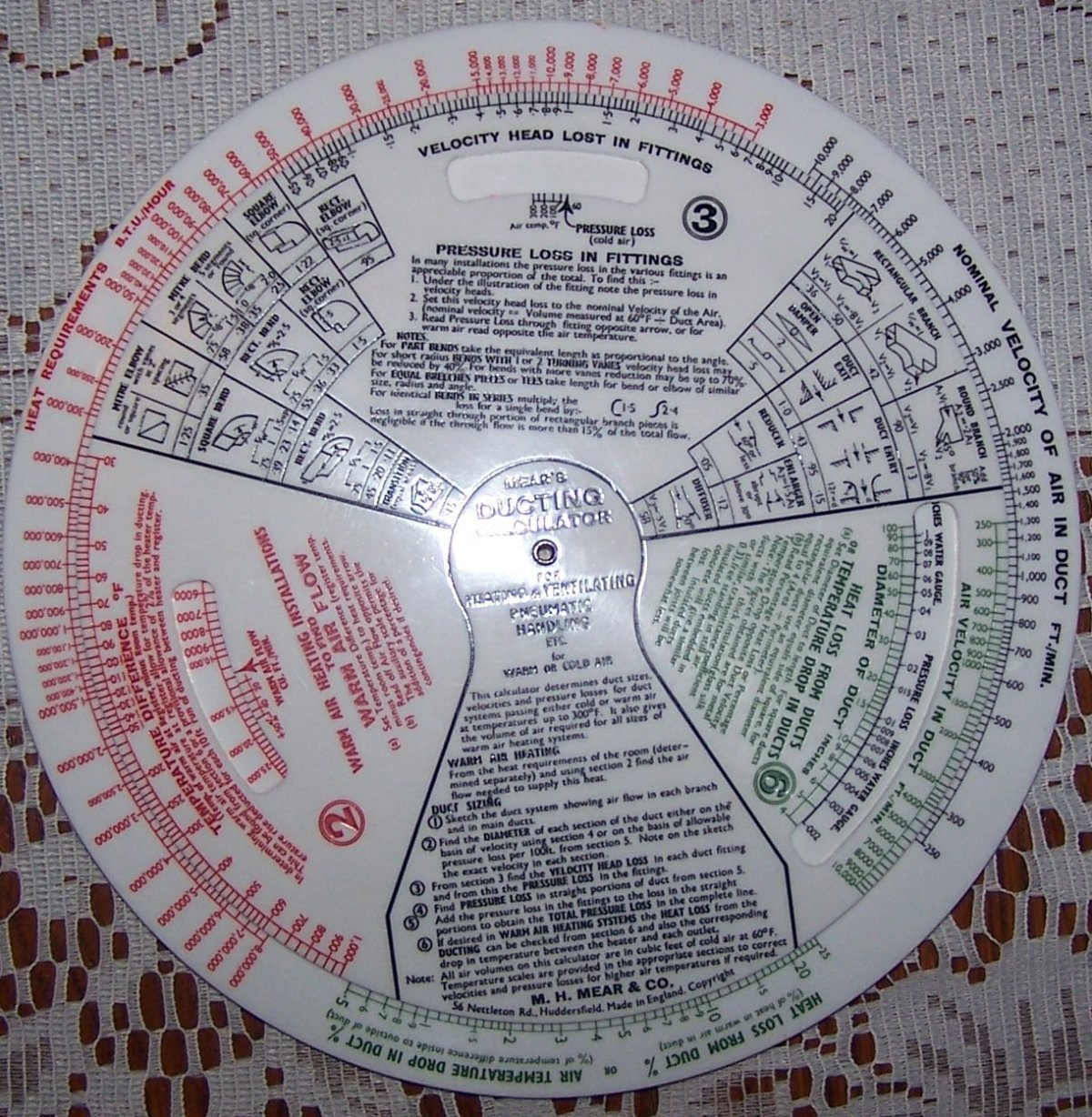Mear's Electric Lighting Calculator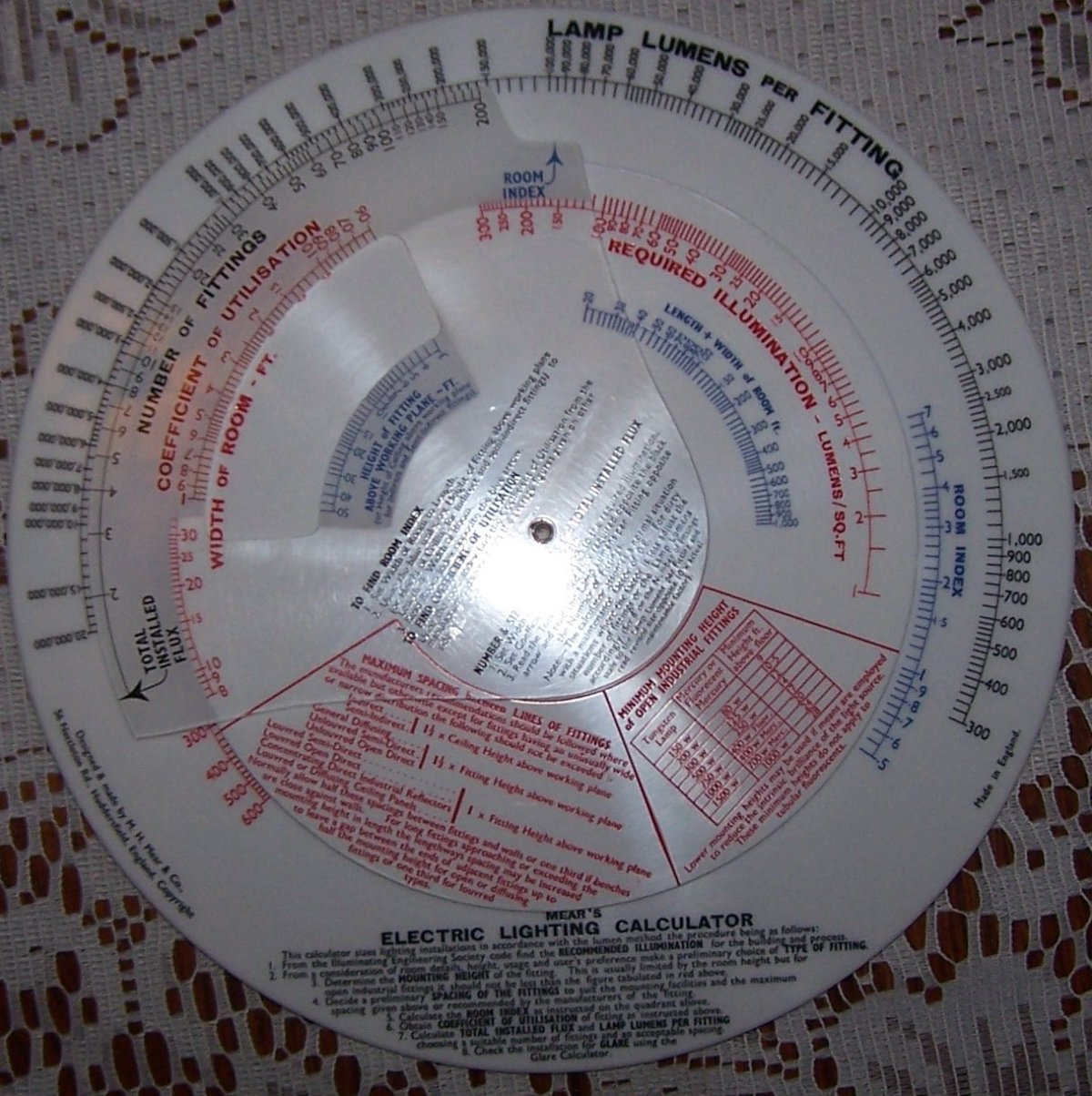Fearns, Mear and Company, Fawcett Extrusion Calculator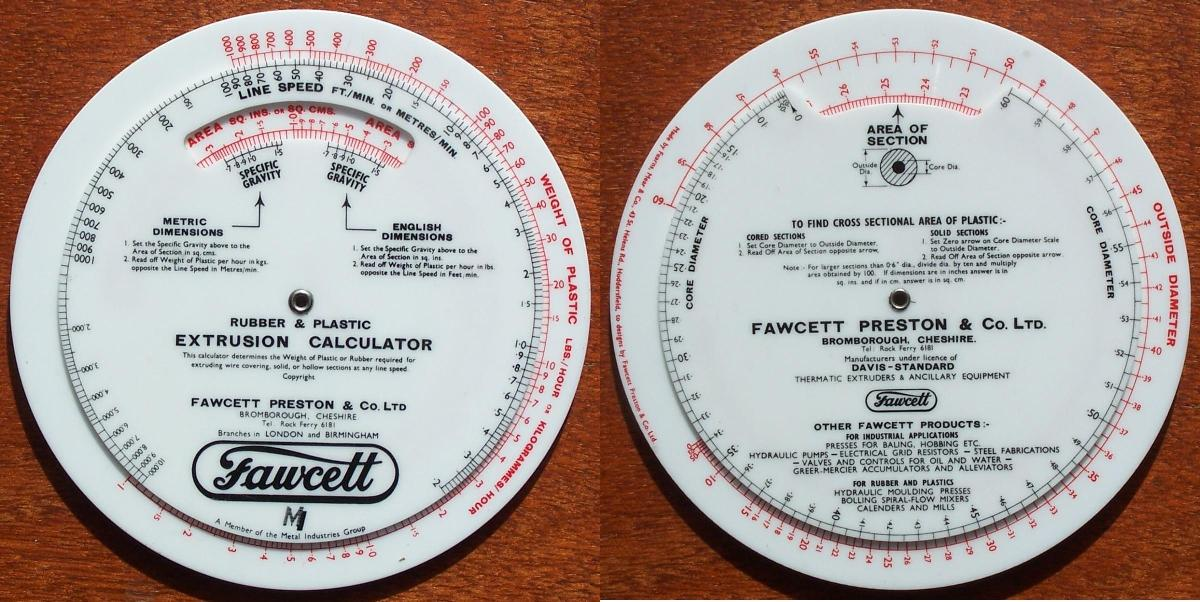Mear's Gas Flow Calculator Metric Model GPMSMear's Gas Flow Calculator Imperial Model G4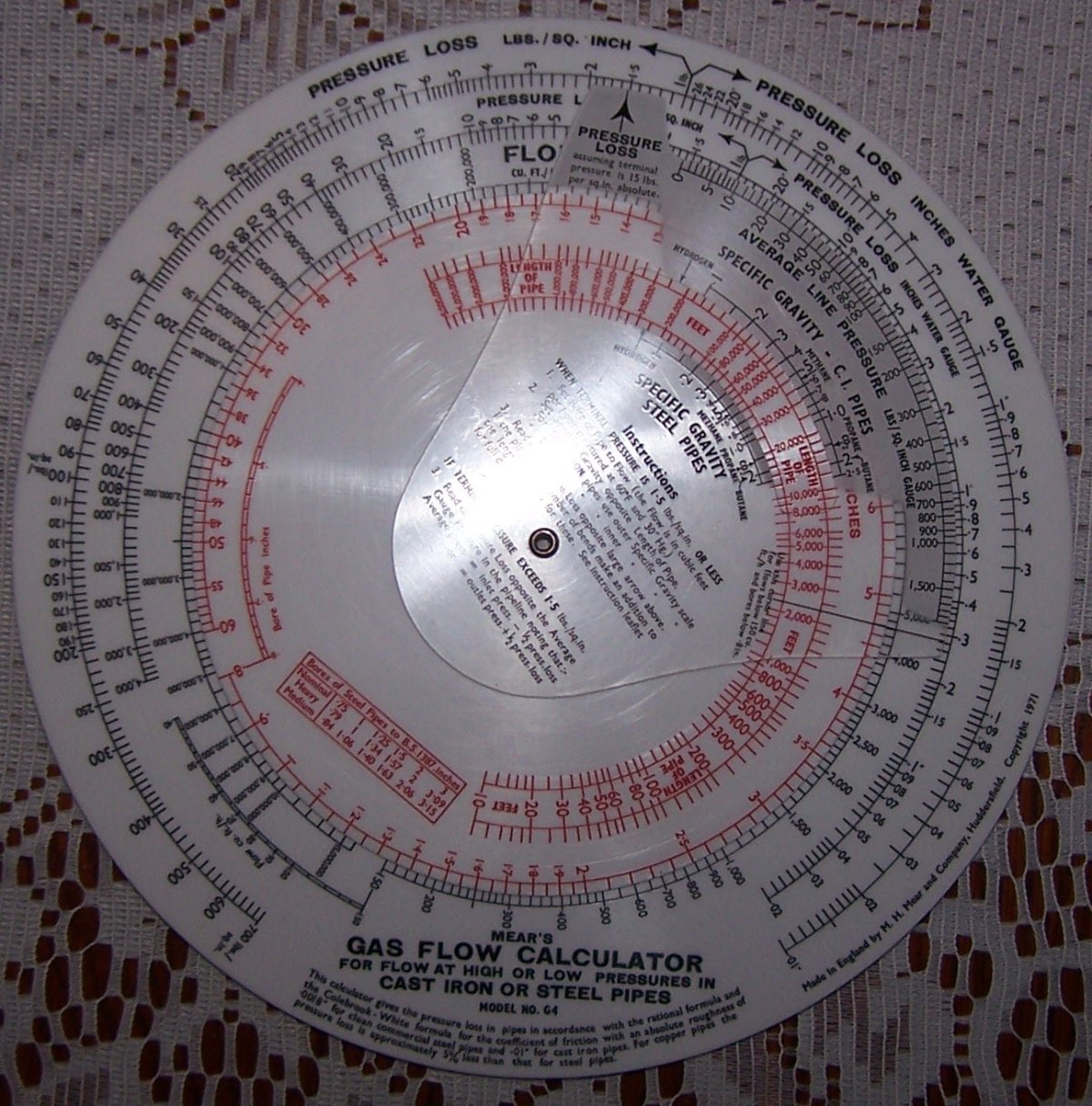Fearn's Gear Rating Computer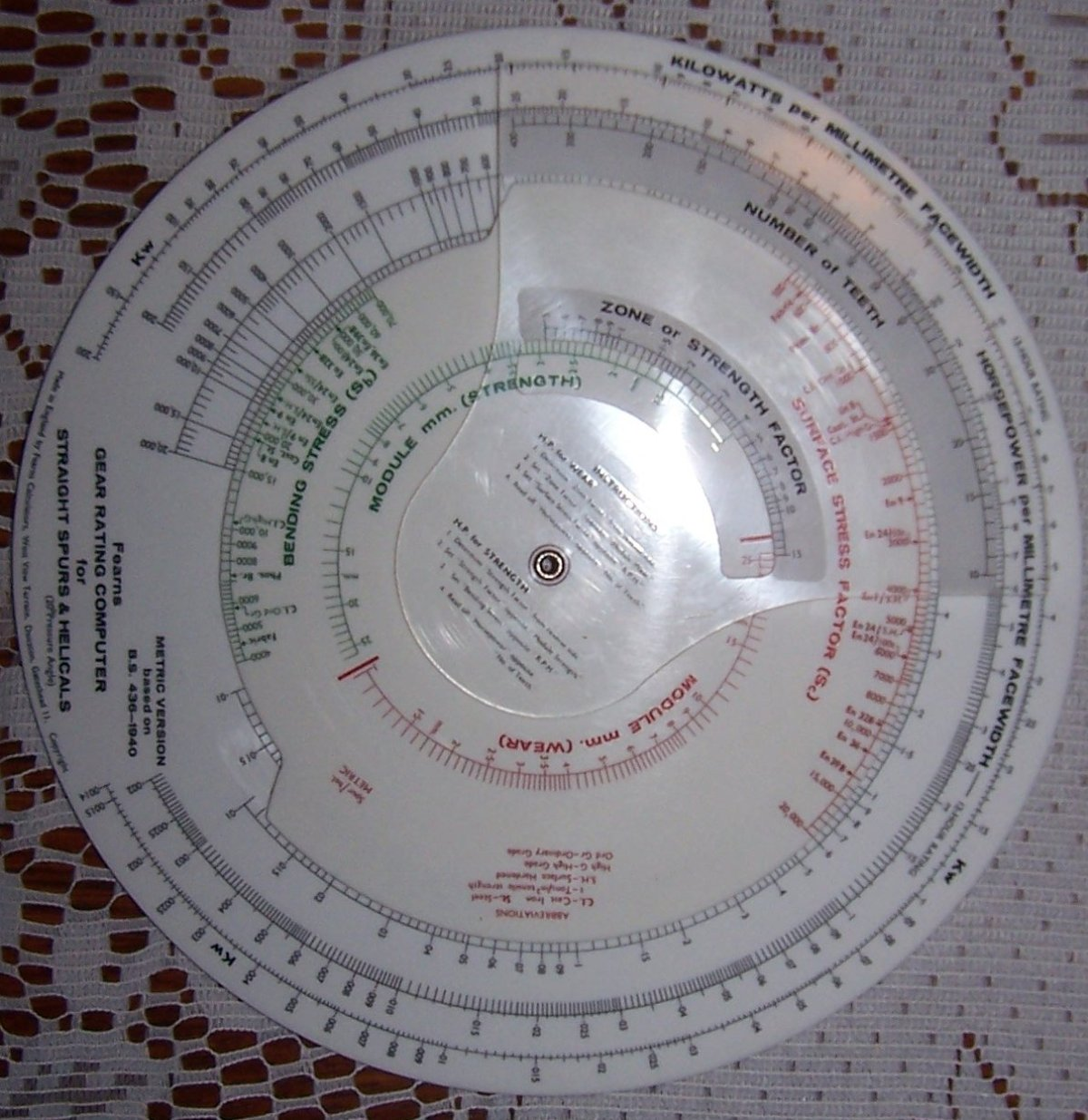Mear's Glare Index Calculator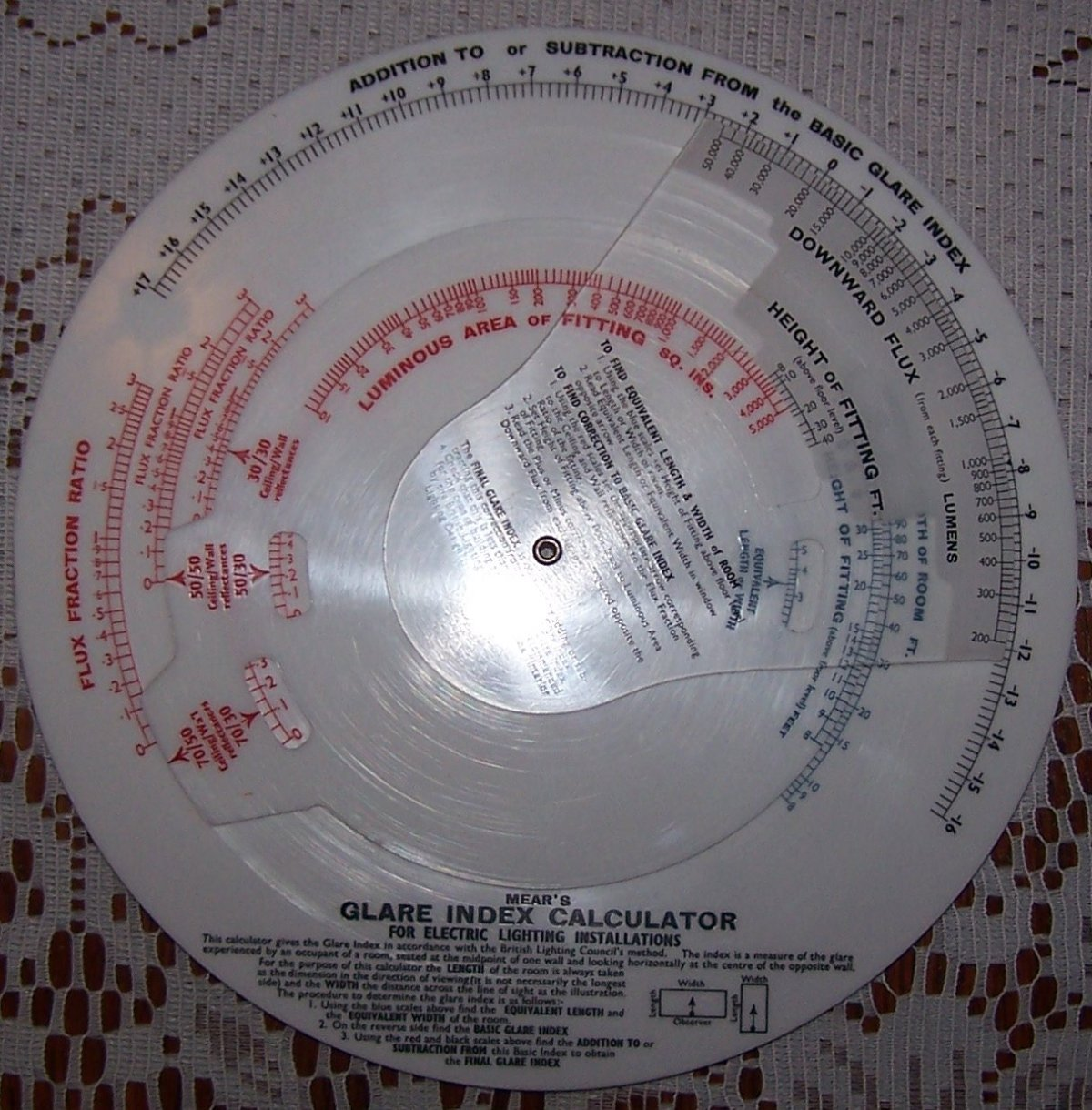Mear's Helical Spring Calculator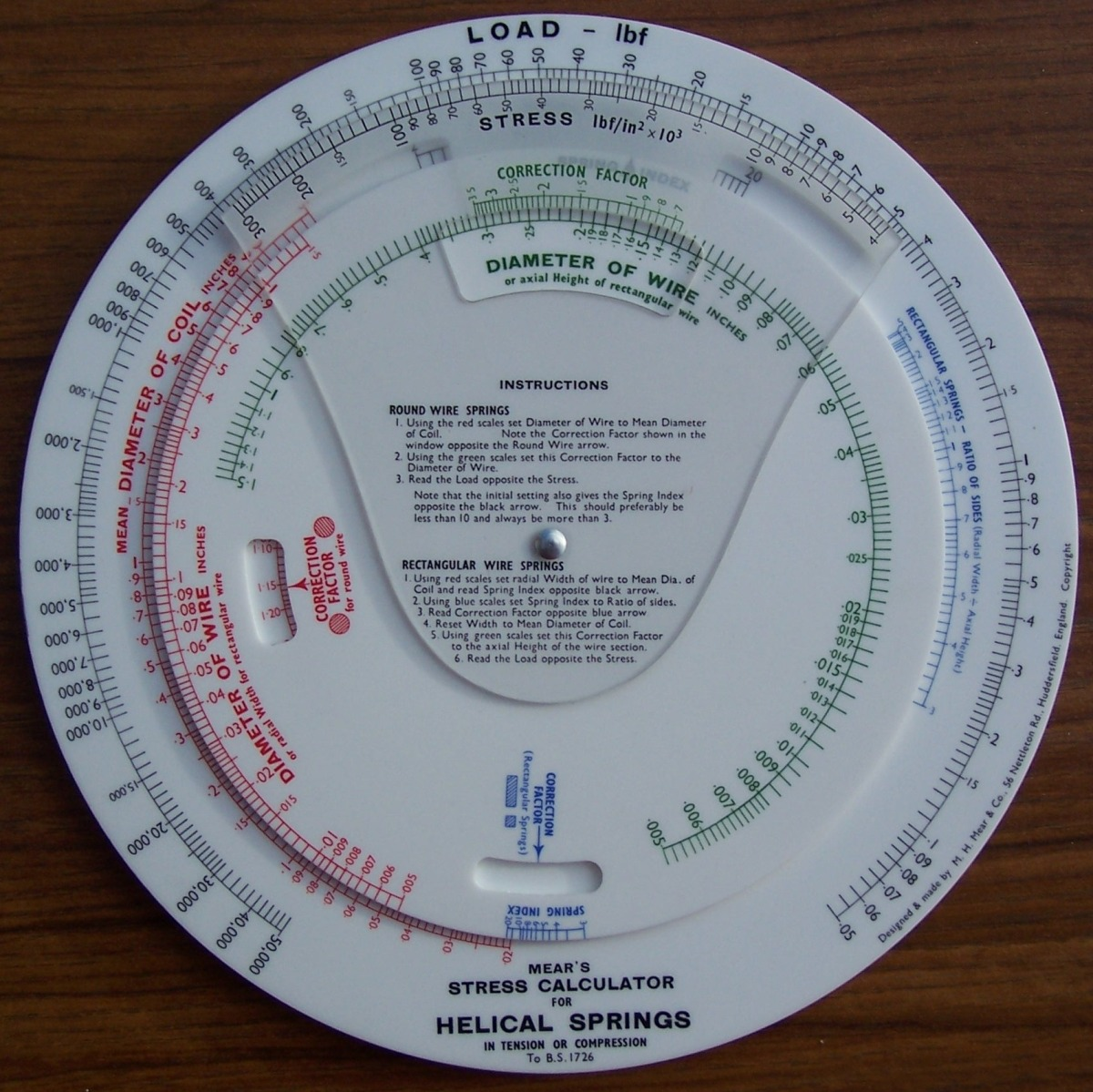Fearns Inches to Millimetres Conversion Calculator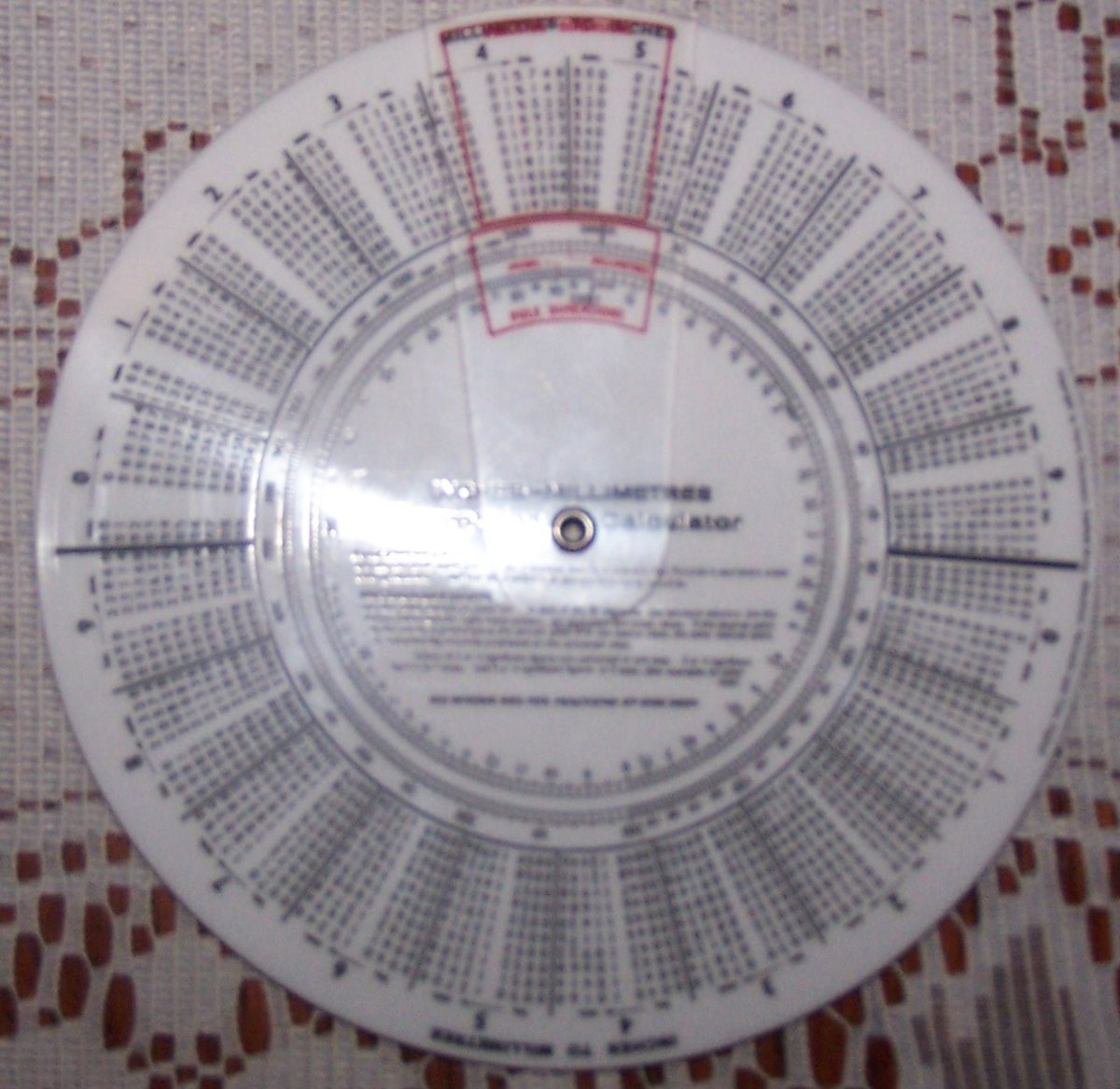Mears Inches to Millimetres Conversion Calculator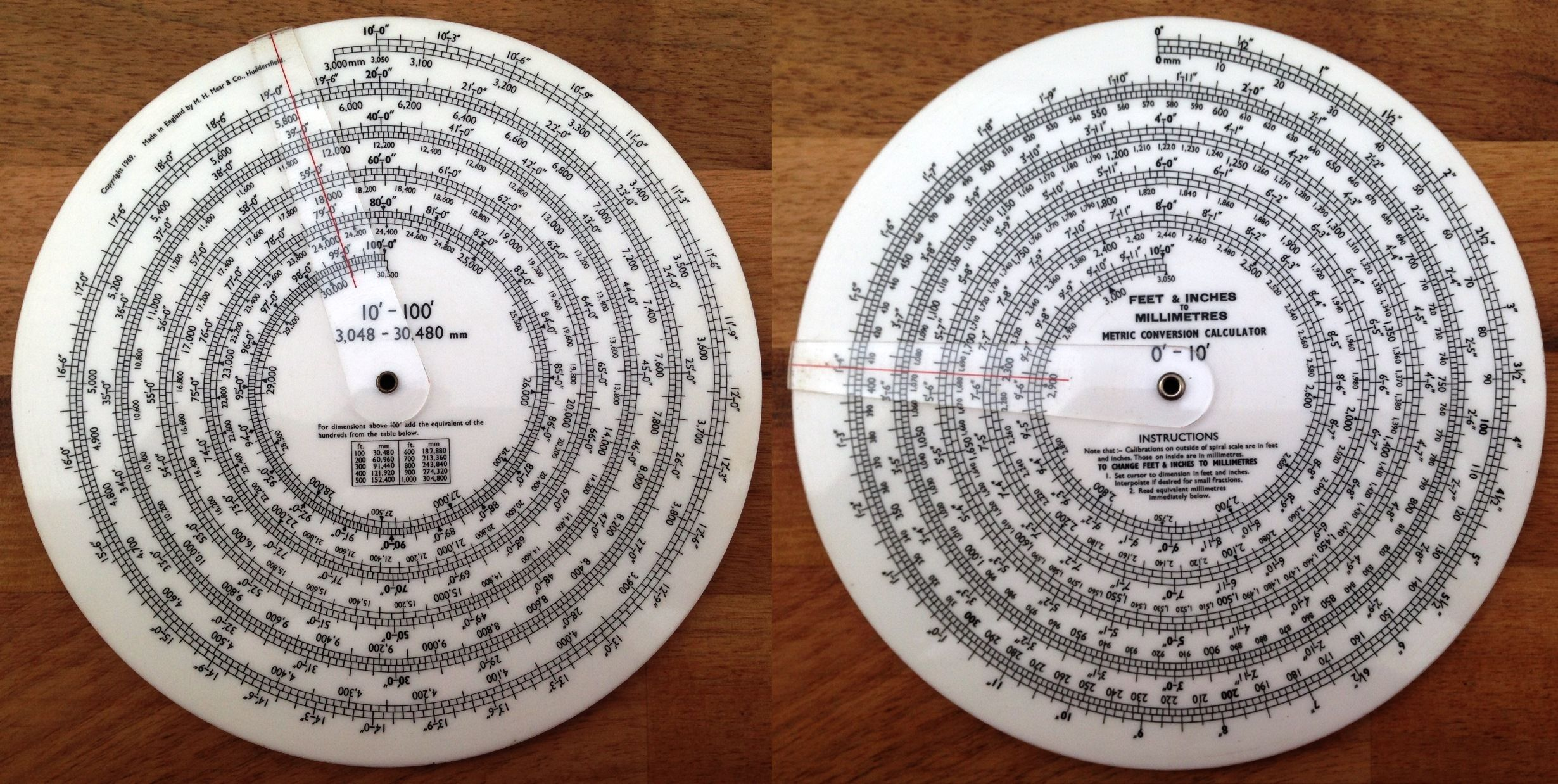Fearn's Inertia Calulator for Rotating Masses Metric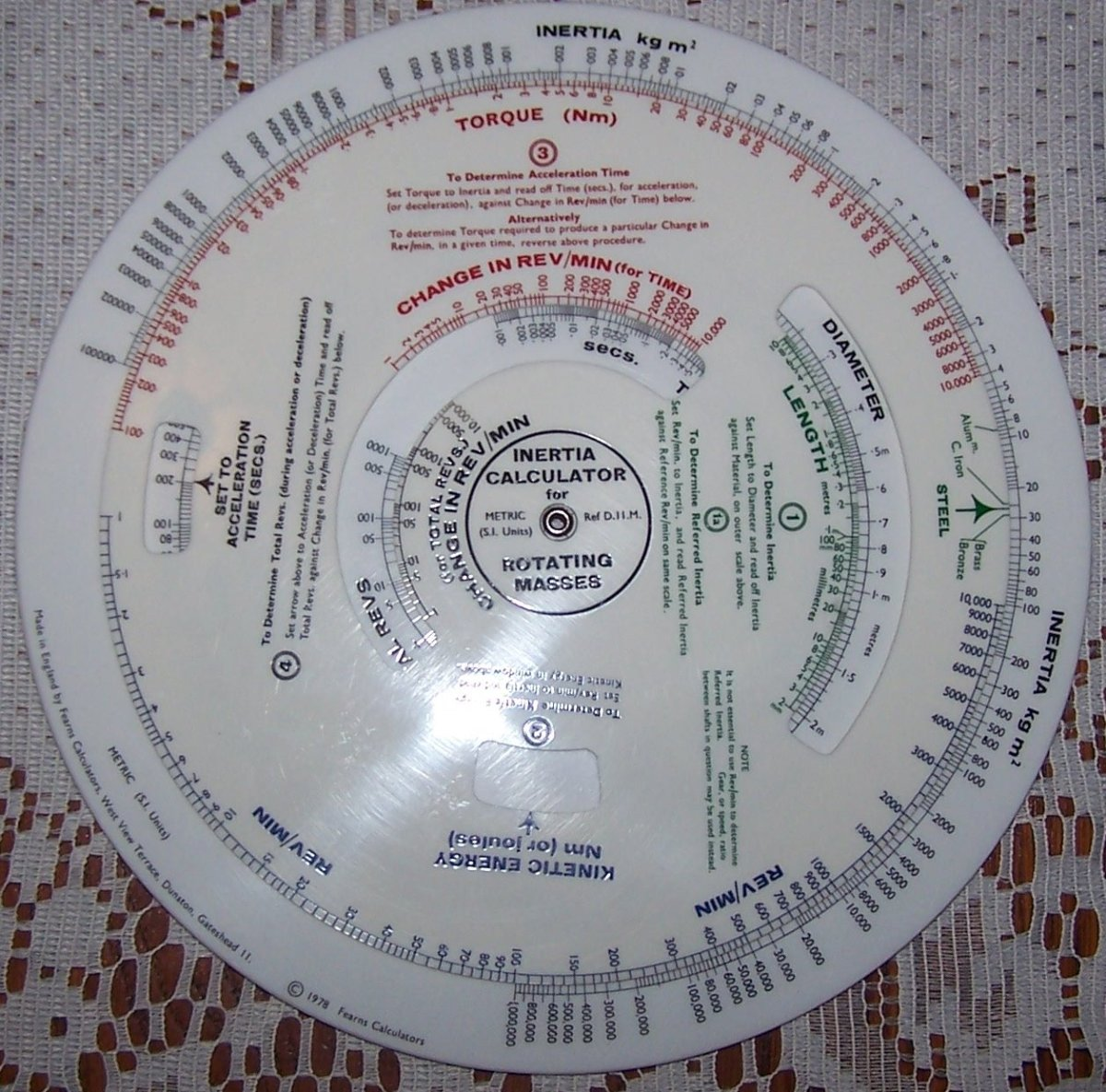Fearn and Mear's Machining Time Calculator for LatheworkMear's Pipe Flow Calculator for Liquids and Gases (Turbulent Flow) Model No.3Fearns Evershed Powerotor Power Calculator.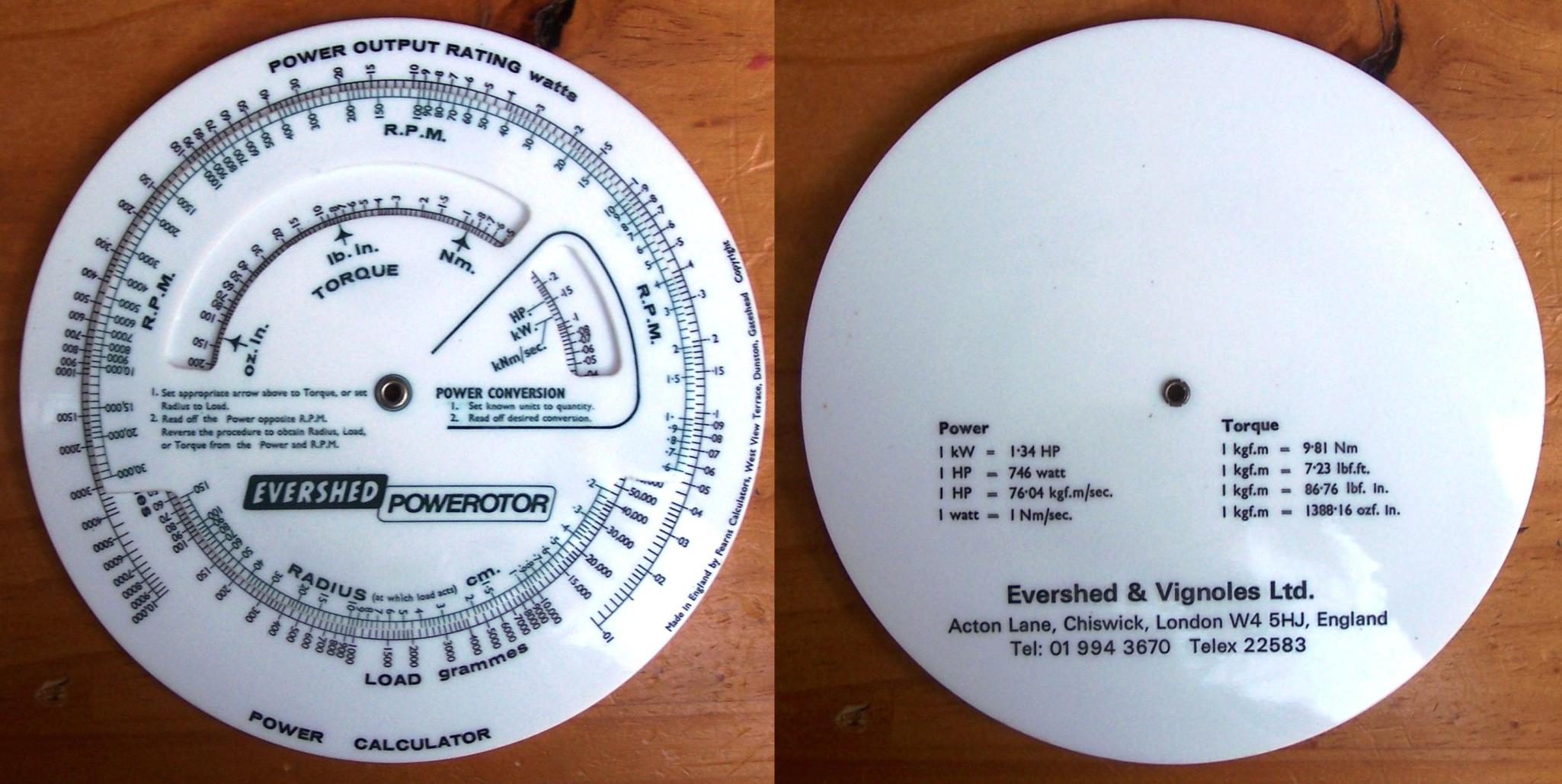Fearn's Presswork Calculator, Model P.42.Fearn's Production Engineer's Calculator, Metric Model P.30.M. For Machine H.P., Batch and Production Times, Speeds, Feeds and Cutting TimesMear's Psychrometric Calculator for Air Coditioning and Meteorology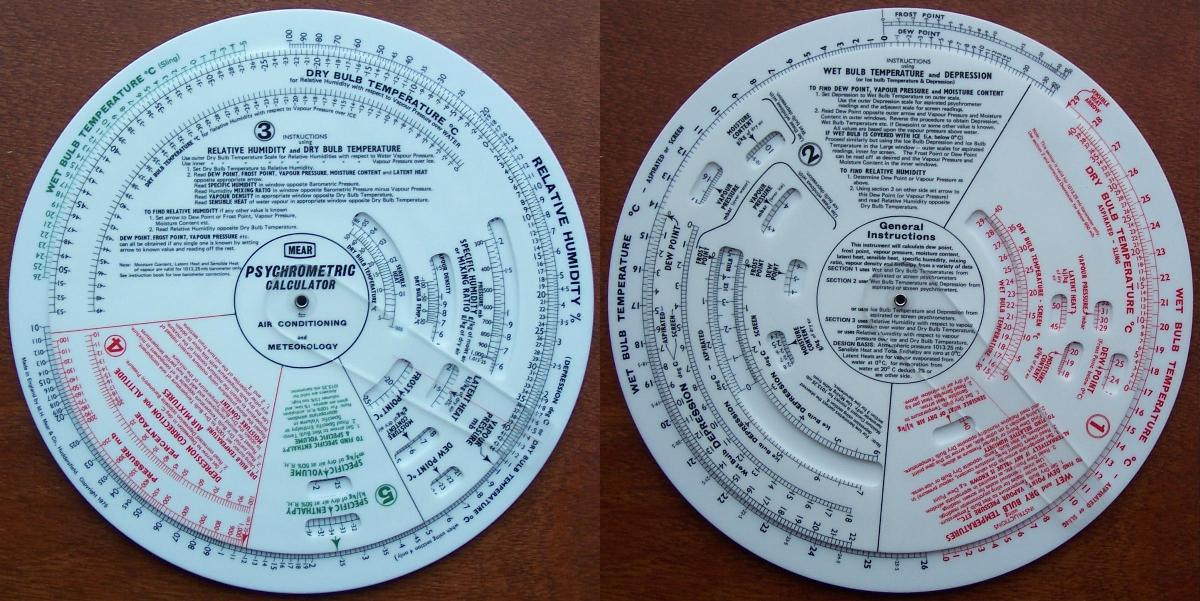Fearn's Re-Order Point Calculator, Model A61Fearn's Shaft Size and Horsepower CalculatorFearn's Slide Rule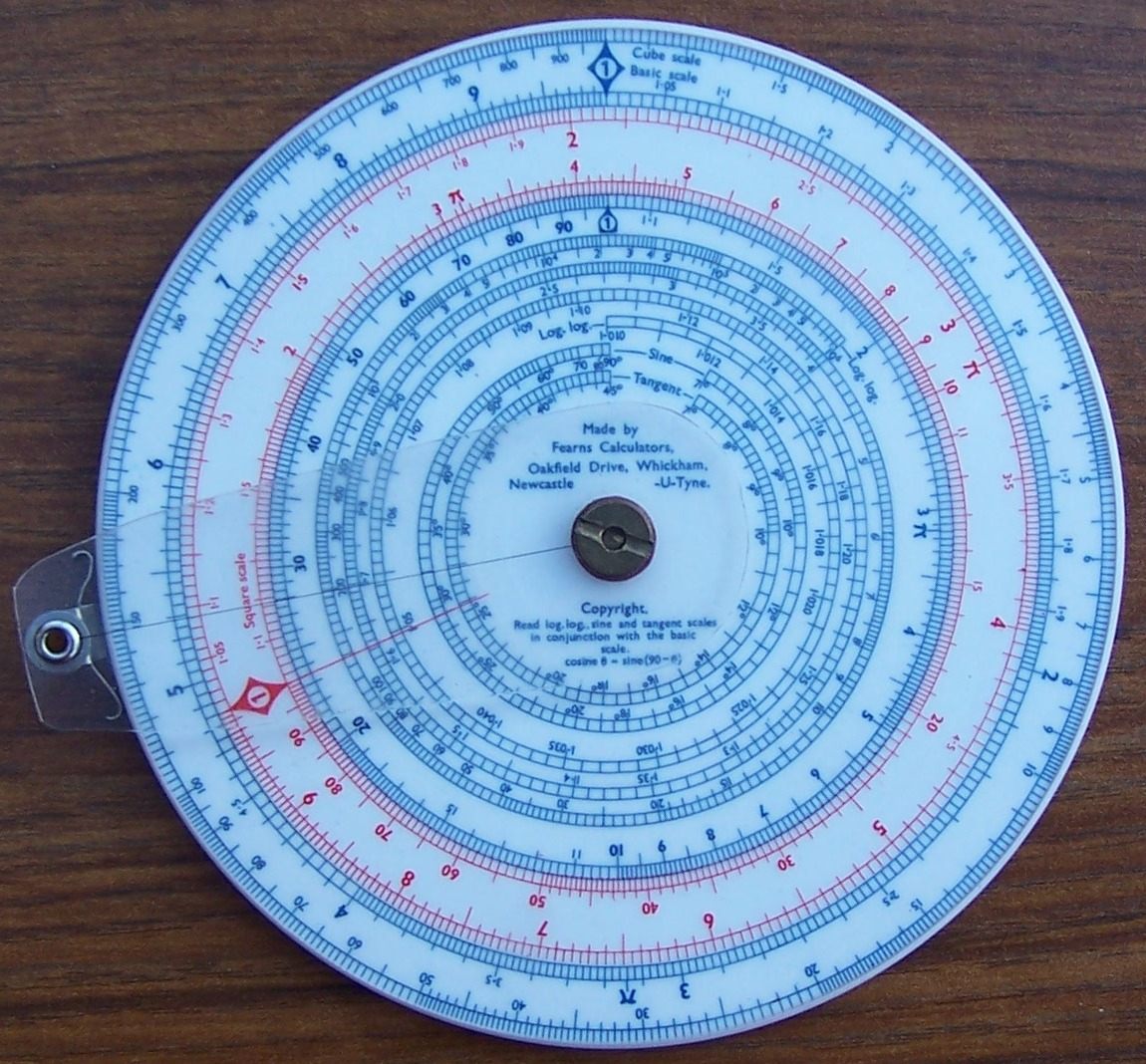Fearn's Open University Slide Rule for course TM281.Mear's Steam Flow Calculator for Flow at Pressure or Vacuum.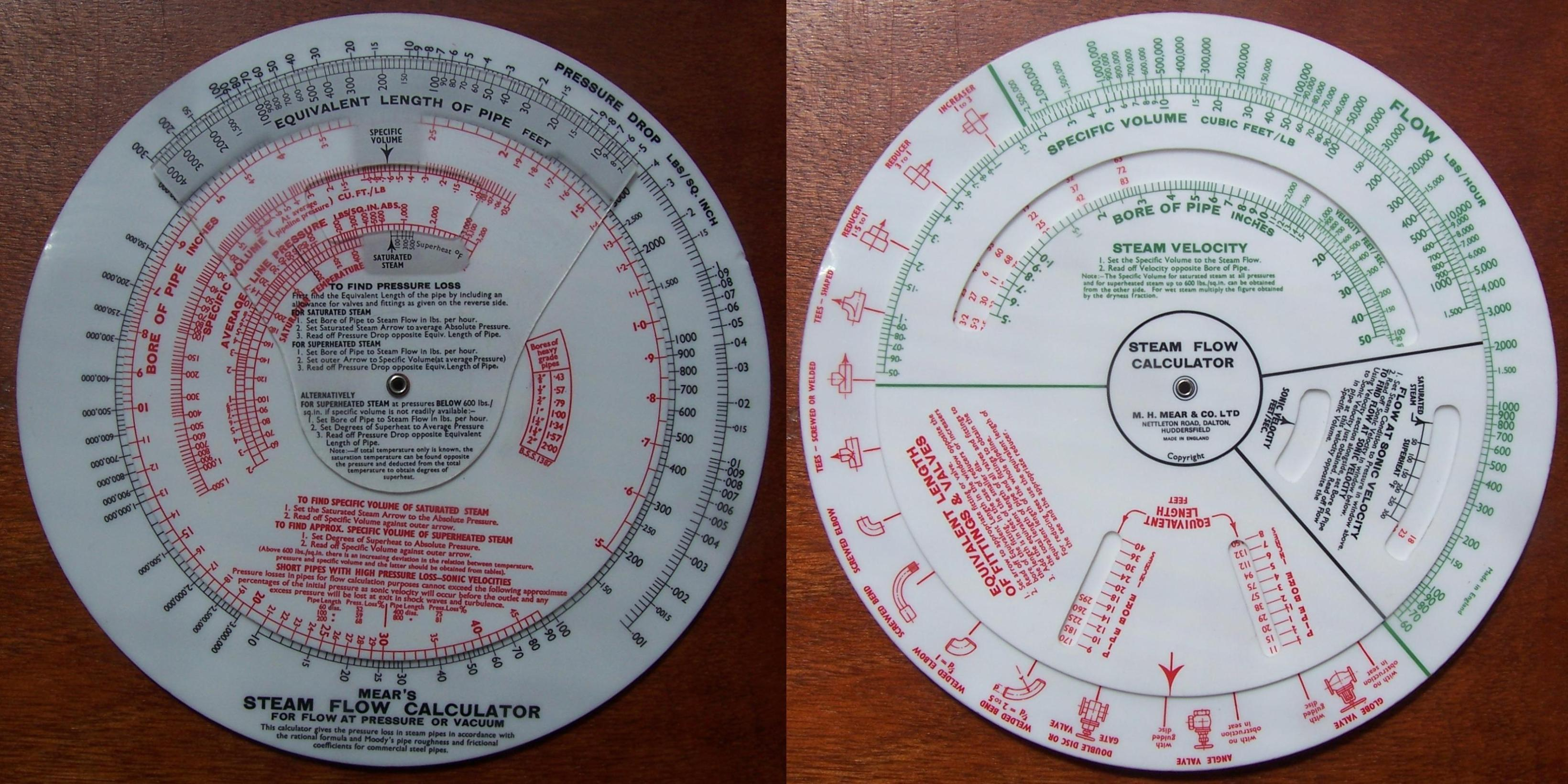Fearn's Tank Capacity CalculatorFearn's Typographer's Calculator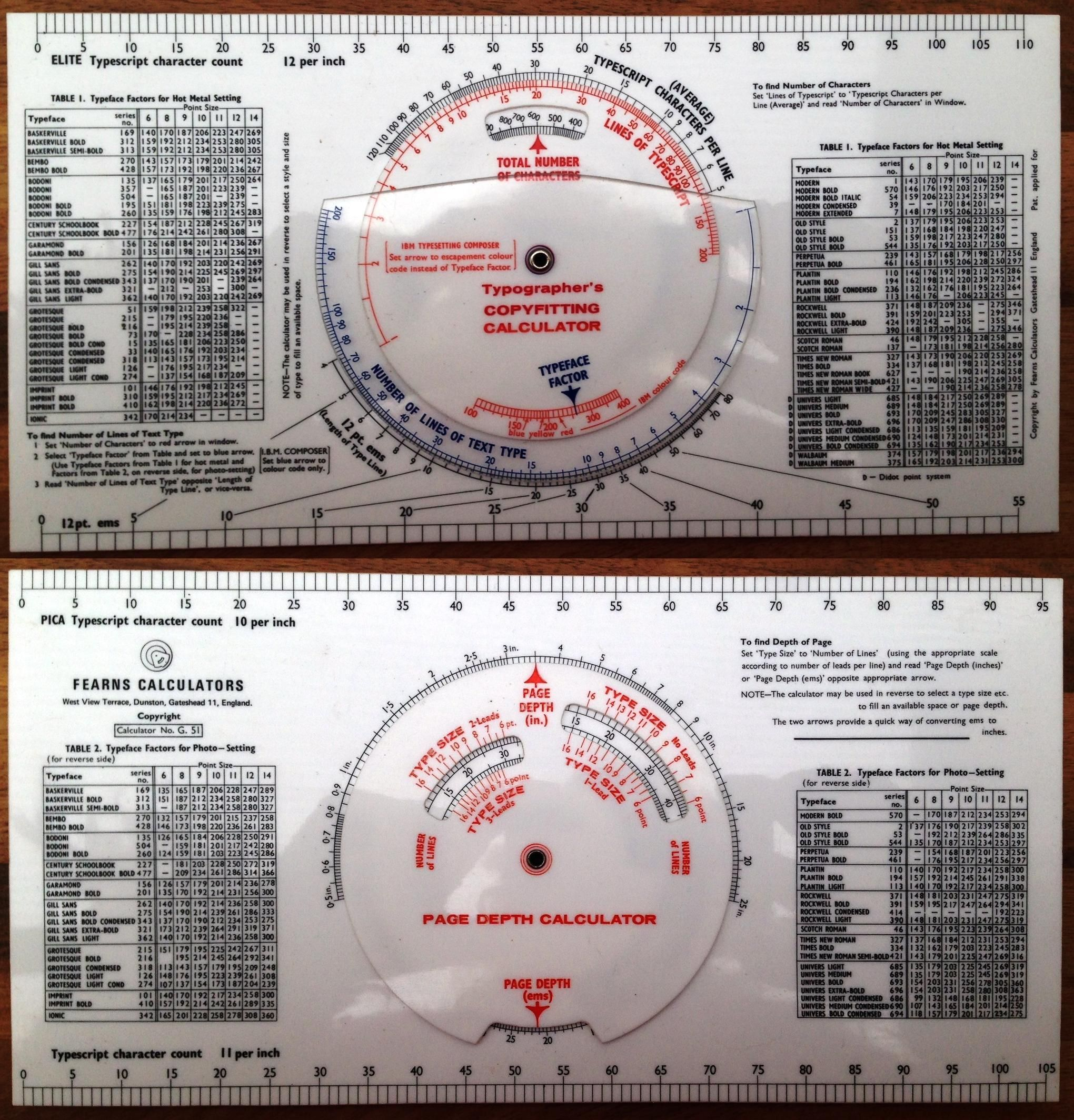Mear's Valve Sizing Calculator for Hydraulic Pressing Operations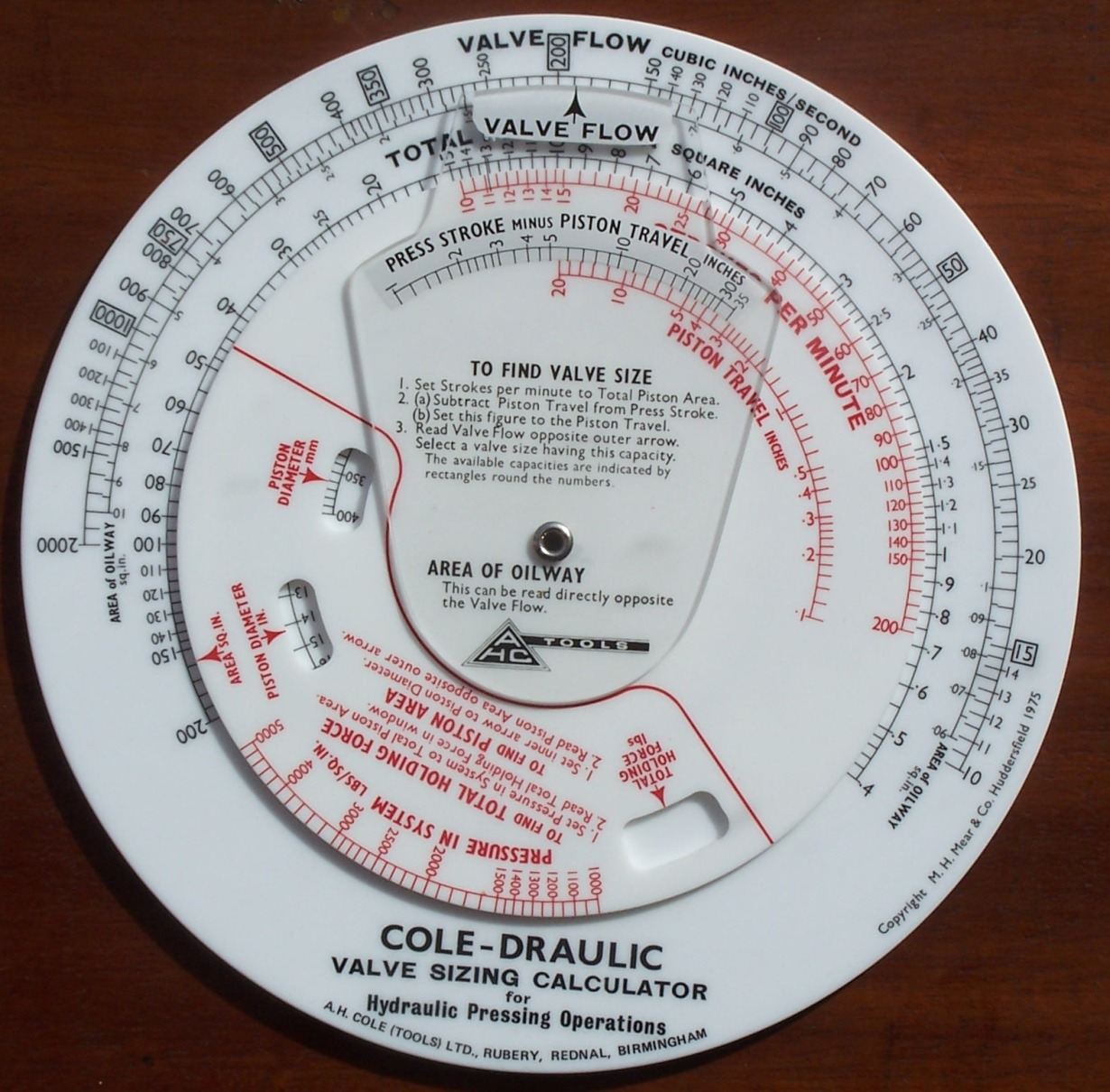Fearn's Vee-Belt Horsepower Calculator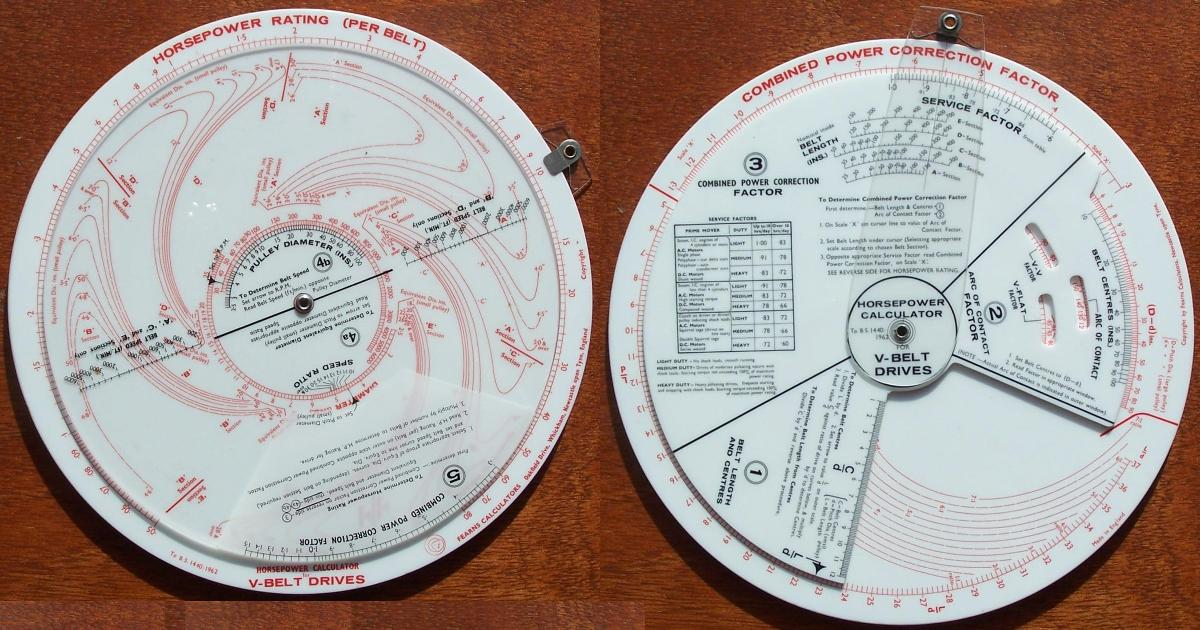Mear's Warm Air Heating Calculator for Domestic InstallationsMear's Weight Calculator for Rolled Steel Sections (Imperial)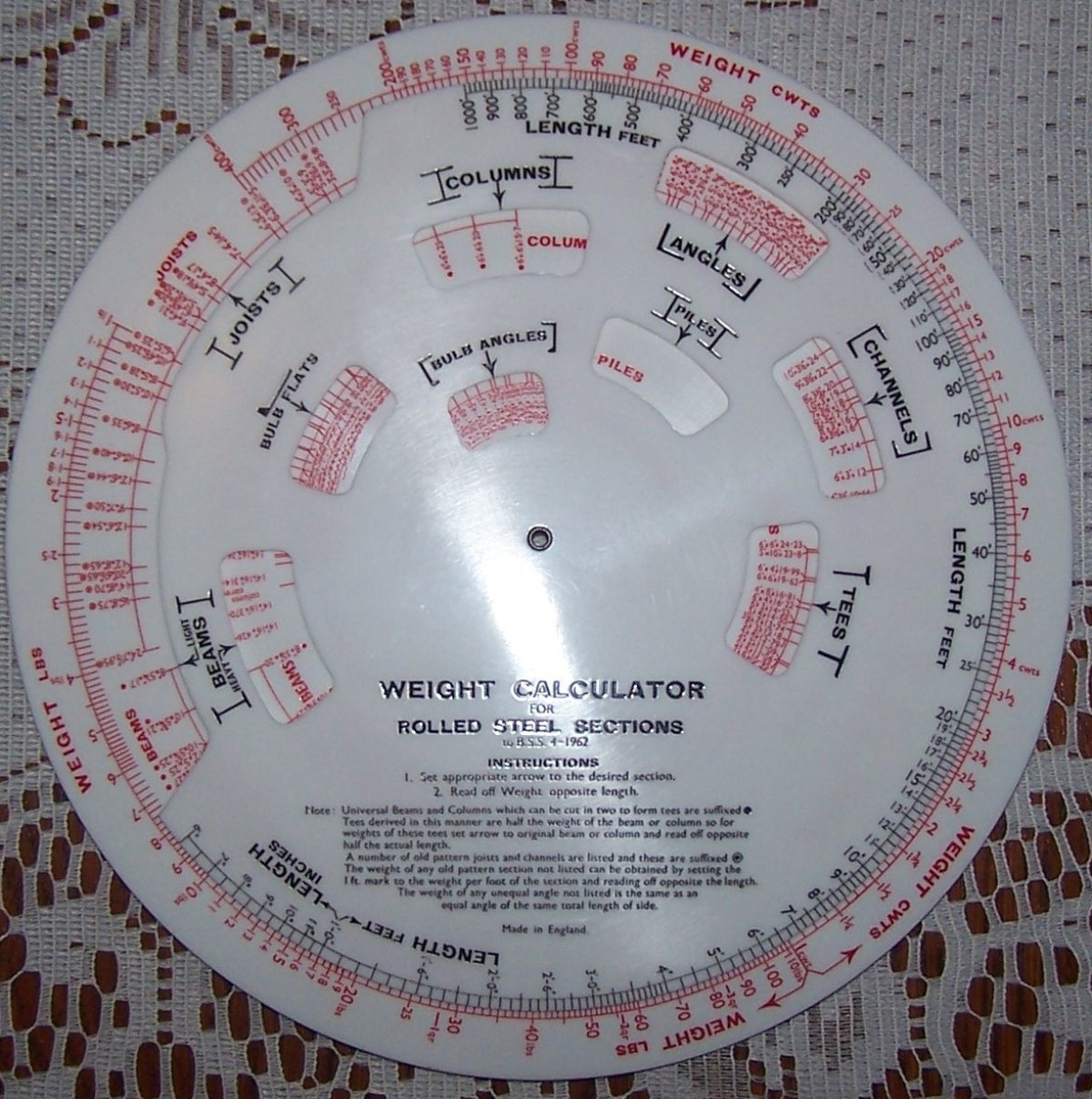Mear's Weight Calculator for Rolled Steel Sections (Metric)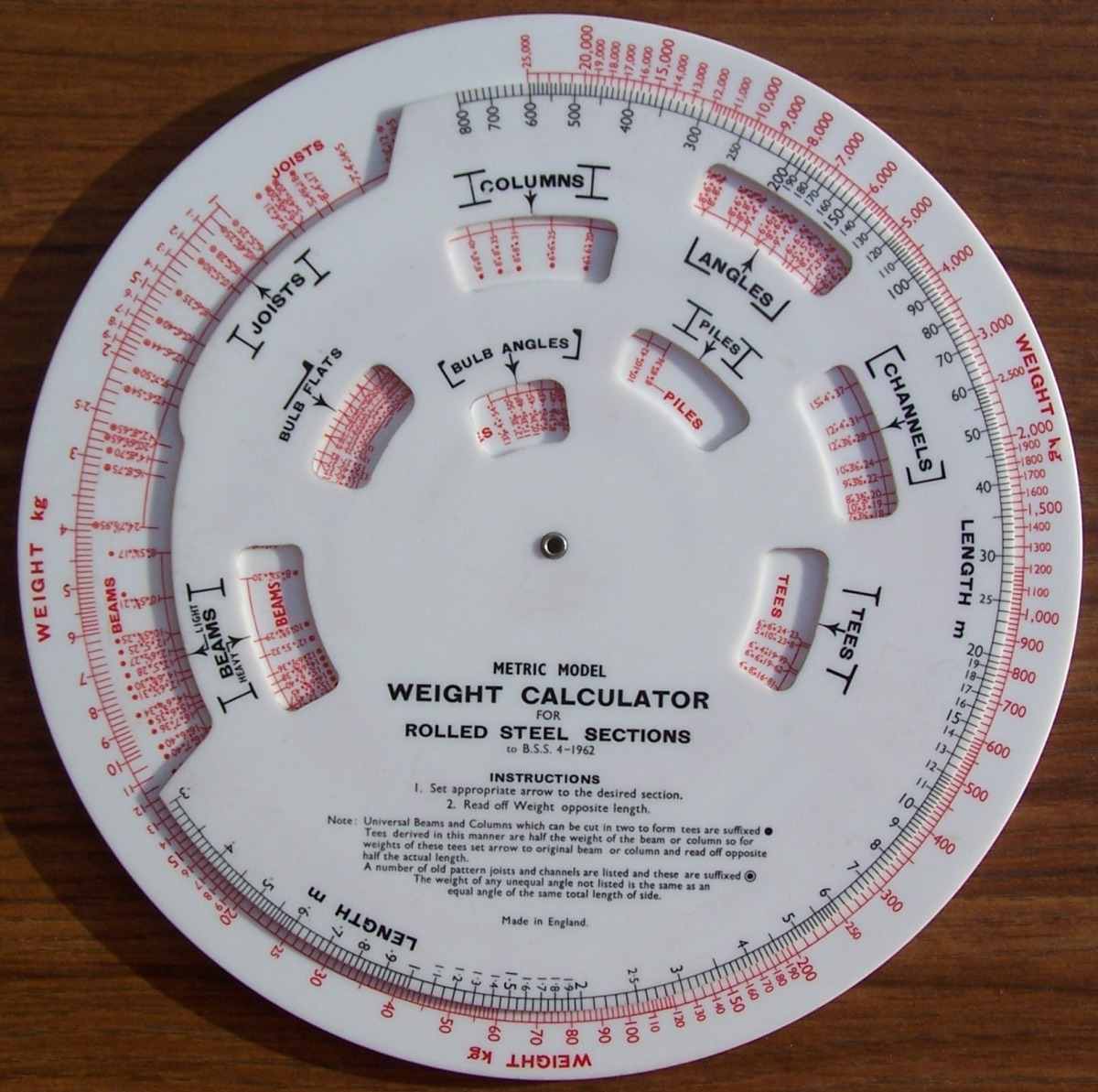Mear's Weight Calculator for Rolled Steel Sections (Imperial) Made for the Consett Iron Company Limited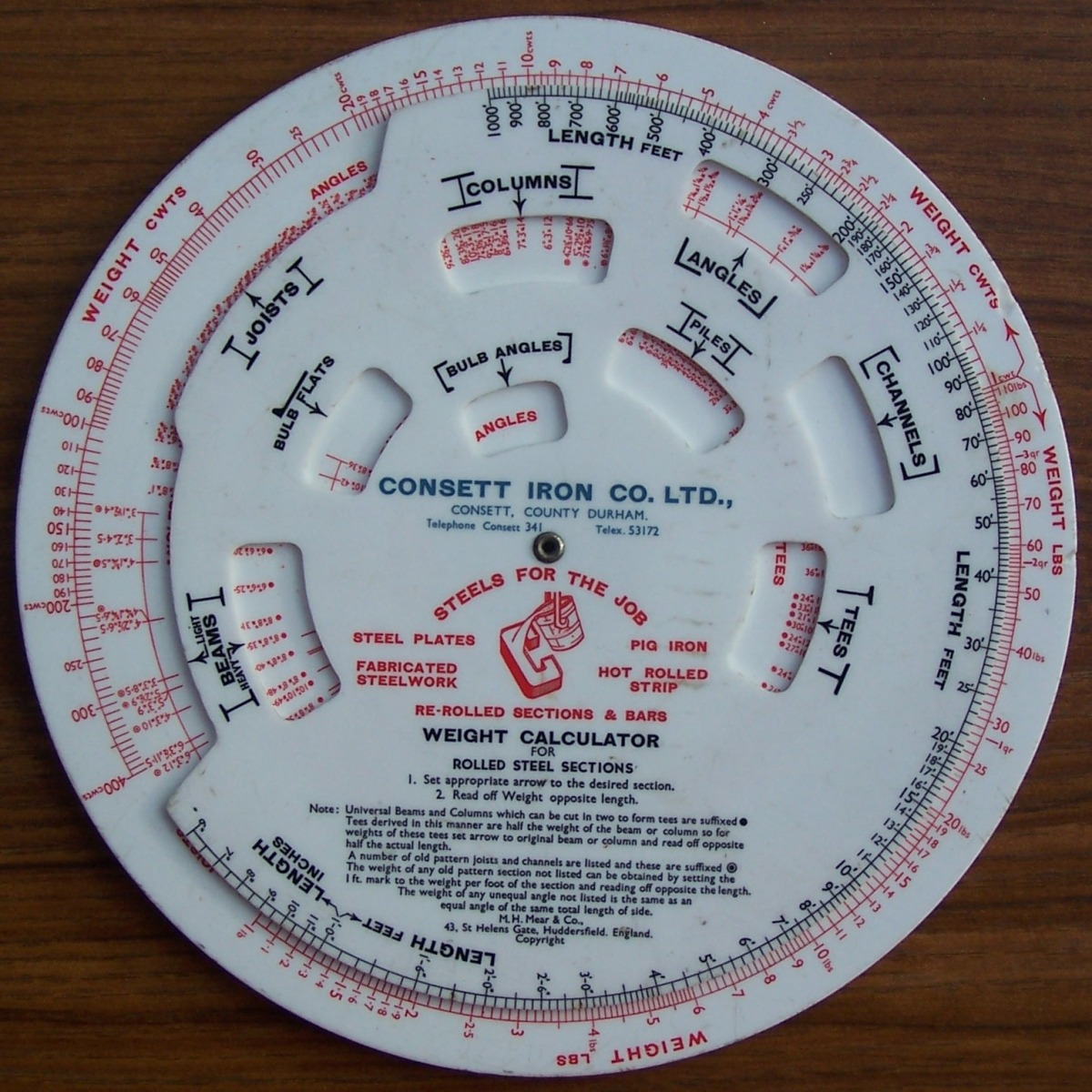Fearn's Weight Calculator for Plates and Sheets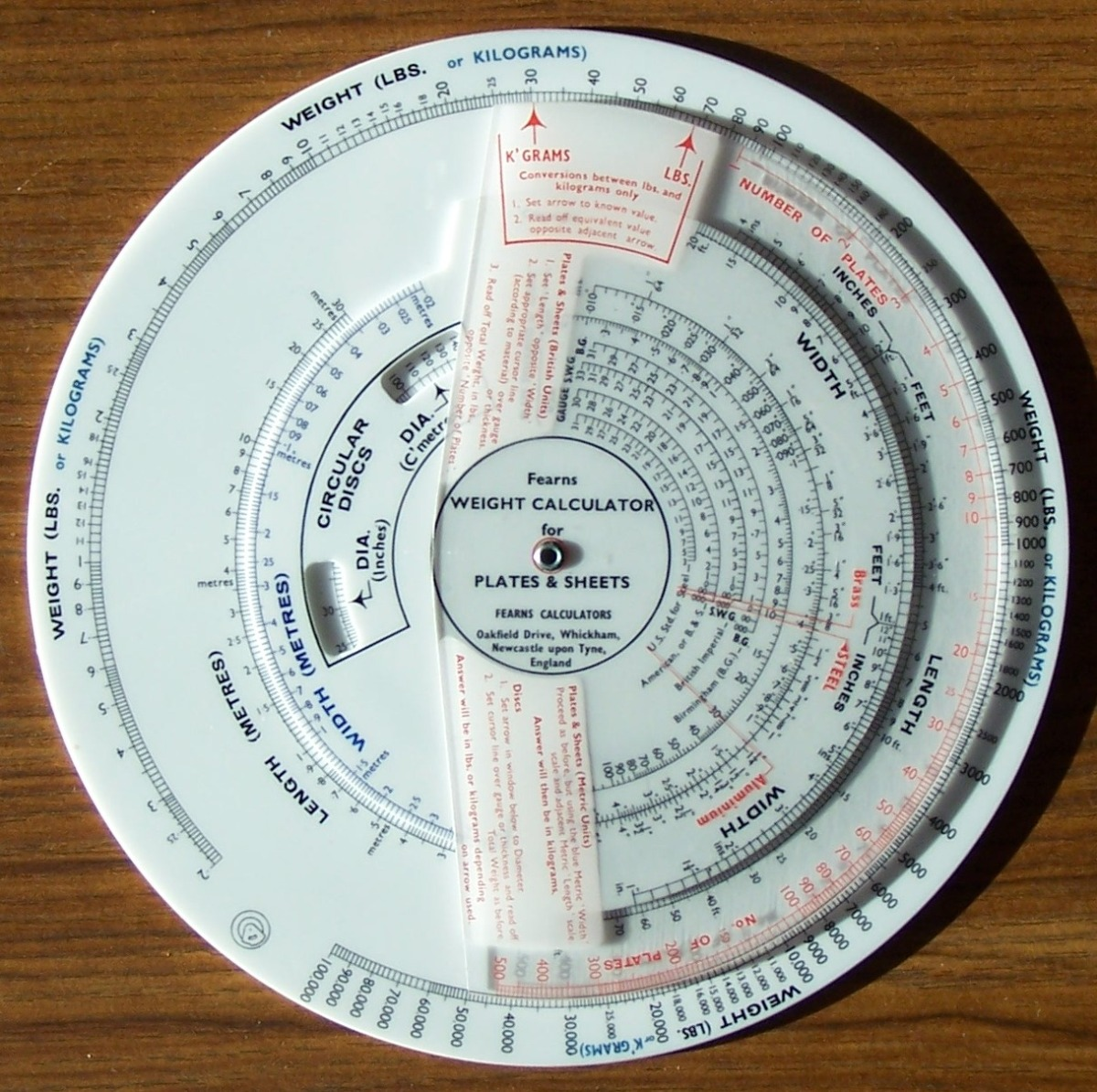Mear's Weight Conversion Calculator, Avoirdupois to Metric